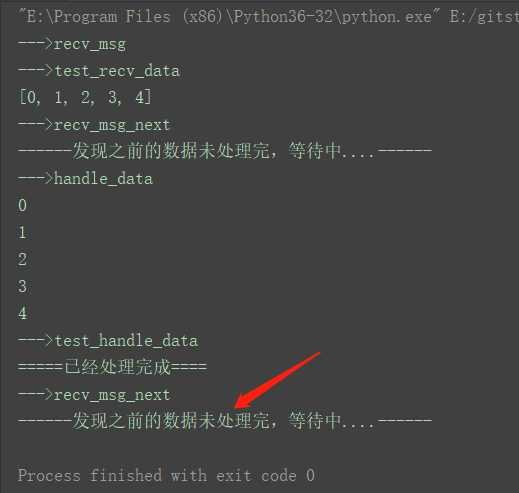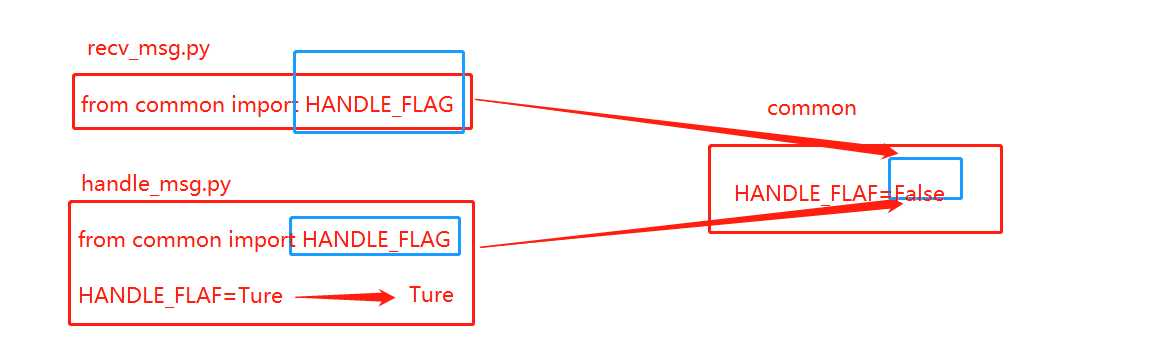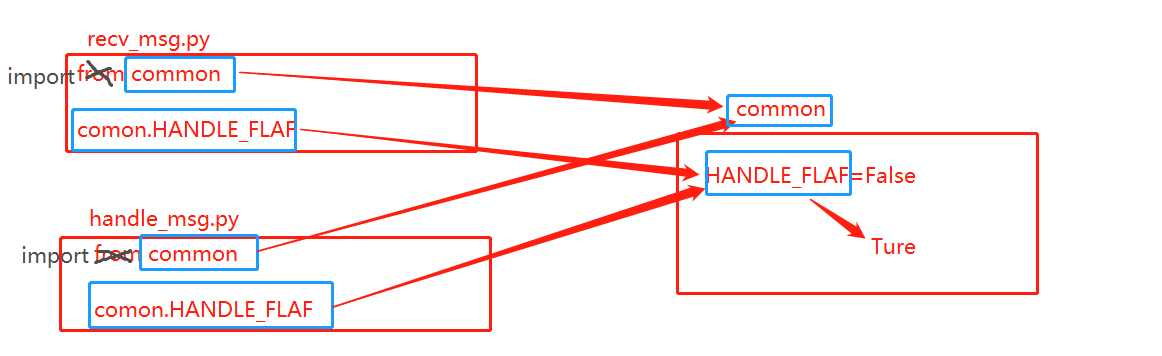# python中多模块导入的注意点

main.py
```from recv_msg import *
from handle_msg import *

def main():
# 1. 接收数据
recv_msg()
# 2. 测试是否接收完毕
test_recv_data()
# 3. 判断如果处理完成，则接收其它数据
recv_msg_next()
# 4. 处理数据
handle_data()
# 5. 测试是否处理完毕
test_handle_data()
# 6. 判断如果处理完成，则接收其它数据
recv_msg_next()

if __name__ == "__main__":
main()```

recv_msg.py

```from common import RECV_DATA_LIST
from common import HANDLE_FLAG
# import common

def recv_msg():
"""模拟接收到数据，然后添加到common模块中的列表中"""
print("--->recv_msg")
for i in range(5):
RECV_DATA_LIST.append(i)

def test_recv_data():
"""测试接收到的数据"""
print("--->test_recv_data")
print(RECV_DATA_LIST)

def recv_msg_next():
"""已经处理完成后，再接收另外的其他数据"""
print("--->recv_msg_next")
if HANDLE_FLAG:
# if common.HANDLE_FLAG:
print("------发现之前的数据已经处理完成，这里进行接收其他的数据(模拟过程...)----")
else:
print("------发现之前的数据未处理完，等待中....------")
```

handle_msg.py

```from common import RECV_DATA_LIST
from common import HANDLE_FLAG
# import common

def handle_data():
"""模拟处理recv_msg模块接收的数据"""
print("--->handle_data")
for i in RECV_DATA_LIST:
print(i)

# 既然处理完成了，那么将变量HANDLE_FLAG设置为True，意味着处理完成
global HANDLE_FLAG
HANDLE_FLAG = True
# common.HANDLE_FLAG = True

def test_handle_data():
"""测试处理是否完成，变量是否设置为True"""
print("--->test_handle_data")
if HANDLE_FLAG:
# if common.HANDLE_FLAG:
print("=====已经处理完成====")
else:
print("=====未处理完成====")```

commom.py

```RECV_DATA_LIST = list()  # 用来存储数据
HANDLE_FLAG = False  # 用来标记是否处理完成```HANDLE_FLAG为什么没有被修改为Ture?python中多模块导入的注意点

(0)
(0)